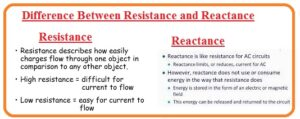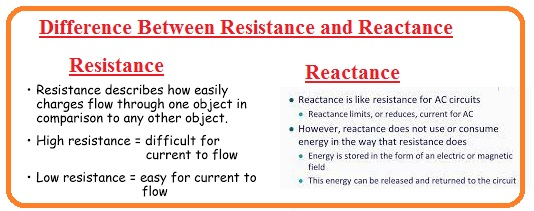Hello guys, I hope you all are doing great. In today’s tutorial, we will have a look at the Difference Between Resistance and Reactance. In electrical circuitry, reactance and resistance are the 2 main parameters that make impedance after coming with each other. The hindrance offered by the current flow in the resistor is called resistance. While reactance is a hindrance in the flow of current through the inductor and capacitor.

In today’s post we will have a detailed look at both resistance and reactance parameters and compare them to find their differences. So let’s get started with Difference Between Resistance and Reactance.

#### Difference Between Resistance and Reactance

Resistance

• The opposition in flow of current through the resistor is called resistance.
• The resistance offered by the material depends on the larger portion that it has.
• The material created with the use of rubber offered a large value of resistance.
• the conductor has less value of resistance
• The material that offered the 0 resistance is called a superconductor
• Its inverse value is electrical conductance.
• It has some features like to friction.
• The resistance value depends on the length of the conductor and the area
• larger length large resistance large area less resistance
• The unknit of conductance is Siemens.
• It is denoted with the R.
• Its formula is R=ρl/A.
• Resistance is exited in both AC and DC circuits.
• It is a real portion of impedance.
• Its value depends on the resistivity, dimension, and temperature of the conductor.
• For resistance the angle between volts and current is similar or there is no phase difference between them.
• Across resistor the part of net power losses.

### What is Ohm’s Law

• According to Ohm law current passing in the substance has proportional relation with the voltage given to that object.

I∝ V

### What is resistivity

• It is a feature of material through which current is passing and also called specific electrical resistance. The inverse of electrical resistivity is called electrical conductivity.
• The unit of resistivity ohm meter.

What is Ohmmeter

• The ohmmeter is a device that is used to find the value of resistance. There is another type of meter called a megohmmeter that is used to find large values of resistance.
• Its measuring unit is ohm

Reactance

• The flow of current through the circuitry has an inductor or capacitor.
• A larger value of reactance caused to make less value of voltage given.
• Reactance is like resistance and has some differences that not lose power like resistance.
• Reactance is used to measure the amplitude and phase of ac.
• With the increment in frequency, there is an increment in inductive reactance
• It is denoted as X.
• inductive reactance is a feature of the inductor and defines the current flow through the inductor
• It is an imaginary portion of impedance.
• Its formula for an inductor is XL=2ΠfL and for a capacitor XC=1/2ΠfC.
• This term is only related to circuitry through which AC current is flowing.
• The circuit component for reactance is an inductor or capacitor.
• Its value depends on the frequency of AC current.
• For reactance, there is a phase difference between volts and current.
• For this element, some part of the power given to the circuit is stored.

### Resistance Vs Reactance

• There is the same behavior shown by resistance to reactance. The circuitry consists of reactance is works like the circuitry creates through zero value reactance.
• These two terms also have some differences in that reactance varies the phase, therefore, the current in the component is moved through 1/4 of the cycle according to the voltage given about the component.
• In pure reactive component, there is no power lost but saved in it. The value of reactance is negative### Resistance Vs Reactance

Resistance Reactance
It restricts the current flow in circuits It causes opposition to an AC current flow in circuits
Its measuring unit is  ohms Its unit is also Ohms
Its value is measured through Ohm’s law Its value is measured through the use of  AC voltage and components the properties
It is directly proportional to voltage about the two points of circuits The complicated quantity consists of capacitive and inductive reactance
It does not depend on the voltage frequency Its value depends on the voltage of the frequency
It does not store the energy It can store energy temporary
It exists in all electrical components it just exists in capacitors and inductors
It uses heat generation and power loss It causes the phase shift of circuits
There are different types of resistors like carbon, wire-wound resistors, and film resistors The main types of capacitors and inductors are electrolytic capacitors and air-core inductors
It is used to control the current flow in circuits It controls the phase shift of ac volts
Resistors have power ratings and tolerance Capacitors have capacitance and inductors have inductance reactances
DC curent not have reactacne AC current has a reactance
multimeter used for resistance measuring frequency meter used
It does not vary the voltage frequency It varies frequency voltage
The total resistance of circuits is the sum of all resistances The total resistance of circuits is the summation of inductive and capacitive reactance
Resistors used in circuits as a current limiter Capacitors used as filter  and timing circuits
Ohm law applicable for resistance Impedance law applied to capacitors and inductors
The decrease of resistance increases the current flow Increasing the value of reactance reduces the curent flow
Its common applications are voltage dividers lighting circuits, power supplies, and It used in radio antennas, resonant circuits,  and transformers
It does not vary the phase angle of the voltage it varies the phase angle of the voltage